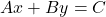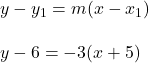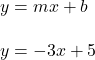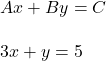## Which of the following is in Standard Form? 1. y – 6 = -3 (x +5) 2. y = -3 (x +5) 3. y = -3x +5 4. 3x + y = 5

Question

Which of the following is in Standard Form?

1. y – 6 = -3 (x +5)
2. y = -3 (x +5)
3. y = -3x +5
4. 3x + y = 5

in progress 0
6 months 2021-08-17T11:01:14+00:00 1 Answers 1 views 0

Equation 4

Step-by-step explanation:

Standard Form is:Option 1 is in point-slope form.

Option 2 is an incomplete point slope equation.Option 3 is in slope-intercept form.This leaves us which option 4, which is in standard form.Option 4 should be the correct answer.

Hope this helps.